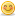##Using Arrays

Hi everyone

I have problem that I am not having much luck with.

I am creating a pie chart and calling this within a command button on another form.

The the command button is clicked, I have a global array within a module

visual basic code
-------------------------------------------------------------------------
Option Explicit

Public Data(0 To 3) As Integer
-----------------------------------------------------------------------

I then call this array. This array is used to sum a field I have within a database i have linked to and then I use the figures from this array to add into 4 lables (lbldata (0 - 3)

THis is all working fine, but what I need to do is to now use the public data (0-3) array detail to populate my pie chart.

What I need to to is to get the total amount and divide each of the 4 totals I have by the total and then multiply 100 to then add into my pie chart.

This is where I am hitting problems.

I think the issue may be because i have already used the data array to populate the lbldata array, I think it needs resetting.

This is what i have

Vb code
--------------------------------------------------------------------------
Sub DrawPieChart()
'Create an array to enable the prgoram to calculate the percentages
'Moves the totals from the NoInStock field totals into the lbl data records
For i = 0 To 3
lblData(i) = Data(i)
Next i
AStart = 0.01
AEnd = 0.01

A = AStart
Debug.Print A
AE = Data(0) * pi / 180 + A
Debug.Print AE
picPieChart.FillColor = CATEGORY1
picPieChart.FillStyle = 0
Debug.Print
picPieChart.Circle (1500, 1200), 1200, vbBlue, -A, -AE

B = AE
Debug.Print B
BE = Data(1) * pi / 180 + B
Debug.Print BE
picPieChart.FillColor = CATEGORY2
picPieChart.FillStyle = 0
picPieChart.Circle (1500, 1200), 1200, vbBlack, -B, -BE

C = BE
Debug.Print C
CE = Data(2) * pi / 180 + C
Debug.Print CE
picPieChart.FillColor = CATEGORY3
picPieChart.FillStyle = 0
picPieChart.Circle (1500, 1200), 1200, vbRed, -C, -CE

D = CE
Debug.Print D
DE = Data(3) * pi / 180 ' This can also be 180 * pi /180
DE = AEnd
Debug.Print DE
picPieChart.FillColor = CATEGORY4
picPieChart.FillStyle = 0
picPieChart.Circle (1500, 1200), 1200, vbYellow, -D, -DE

End Sub

------------------------------------------------------------------------

I tried to add before the pie chart building

VB code
----------------------------------------------------------------------
'Create the totals for the pie chart using the data array from the module

Dim Percentage(0 - 3)

For i = 0 To 3
total = Data(i) + total
Next

For i = 0 To 3
Percentage(i) = Data(i) / total
------------------------------------------------------------------------
To get the calculations but the error I got initally was
Percentage(0 - 3) As String - highlighted with error range has no values.

I am not sure what the best approach is. would it be to reset the Data array? Or is thre a different approach I should be taking.

i have tried doing to get the figures i need
AE = Data(0) / Data(i) * 100 * pi / 180 + A

But this does not work either.

I am sure there is a imple soultion for my problem and I would appreciate it of anyone can assist me with this one.

Thanks

Tracey